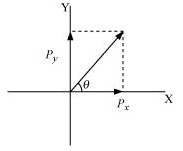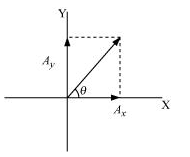## Pages

### Motion in a plane NCERT Solutions Class 11 Physics - Solved Exercise Question 4.22

Question 4.22:

i and j are unit vectors along x and y axis respectively. What is the magnitude and direction of the vectors i + j, and ij ? What are the components of a vector A= 2i + 3j along the directions of i + j and ij? [You may use graphical method]

Solution:

Consider a vector P, given as:
P = i + j
Px i + Py j = i + j

On comparing the components on both sides, we get:
Px = Py = 1
|P| = ( Px2 + Py2 )1/2
= (1+1)1/2
= √2    ....(i)
Hence, the magnitude of the vector i + j is √2
Let θ be the angle made by the vector P, with the x-axis, as shown in the following figure.∴ tan θ = ( Py / Px )
θ = tan-1 (1 / 1) = 450 .......(ii)
Hence, the vector i + j makes an angle of 450 with the x-axis.
Let Q = i + j
Qx i - Qy j = i - j
Qx = Qy = 1
|Q|  = (Qx2 + Qy2)1/2 = √2     .... (iii)
Hence, the magnitude of the vector i - j is √2
Let θ be the angle made by the vector |Q|, with the x- axis, as shown in the following figure.∴ tan θ = (-Qy / Qx)
θ = tan-1 (-1/1) = -450     ......(iv)
Hence, the vector i - j makes an angle of -450 with the x-axis.
It is given that:
A = 2i + 3j
Ax i + Ay j = 2i + 3j
On comparing the coefficients of i and j, we have
Ax = 2 and Ay = 3
|A| = (22+32)1/2 = (13)1/2
Let Ax make an angle θ with the x-axis, as shown in the following figure.∴ tan θ = (Ay / Ax)
θ = tan-1 (-3/2)  =  56.310
Angle between the vectors (2i + 3j) and (i + j), θ' = 56.31 - 45 = 11.310
Component of vector A, along the direction of P, making an angle θ'
= (A Cos θ') P^ = (A Cos 11.31) (i + j) / √2
= √13 X 0.9806 (i + j) / √2
= 2.5 (i + j)
= 5 / √2   ........(v)
Let θ" be the angle between the vectors (2i + 3j) and (i - j).
θ" = 45 + 56.31 = 101.310
Component of vector A, along the direction of Q, making an angle θ"
= (A Cosθ")Q = (A Cosθ") (i - j) / √2
= √13 Cos (901.310) (i - j) / √2
= -0.5 (i - j)
= -5 X √2 / 10
= -1 / √2     ....(vi)